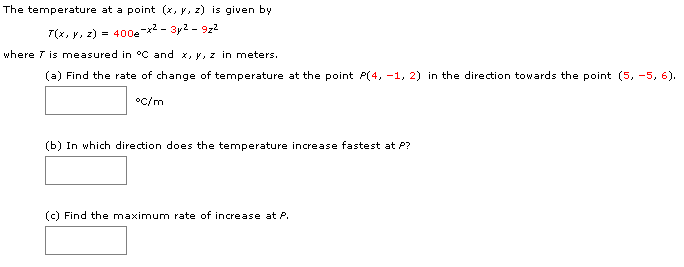# The temperature at a point (x, y, z) is given by400ex-3y2- 9z2--_(x, y, z)where T is measured in °C and x, y, z in meters(a) Find the rate of change of temperature at the point P(4, -1, 2) in the direction towards the point (5, -5, 6)oc/m(b) In which direction does the temperature increase fastest at P?(c) Find the maximum rate of increase at P.

Question
2 views

The temperature at a point

(x, y, z)

is given by

T(x, y, z) = 400e−x2 − 3y2 − 9z2

where T is measured in °C and

x, y, z

in meters.help_outlineImage TranscriptioncloseThe temperature at a point (x, y, z) is given by 400ex-3y2- 9z2 --_ (x, y, z) where T is measured in °C and x, y, z in meters (a) Find the rate of change of temperature at the point P(4, -1, 2) in the direction towards the point (5, -5, 6) oc/m (b) In which direction does the temperature increase fastest at P? (c) Find the maximum rate of increase at P. fullscreen
check_circle

Step 1

As the question doesnt specifies the part to be answered so answering the part 'a' of the question here.

The...

### Want to see the full answer?

See Solution

#### Want to see this answer and more?

Solutions are written by subject experts who are available 24/7. Questions are typically answered within 1 hour.*

See Solution
*Response times may vary by subject and question.
Tagged in

### Math